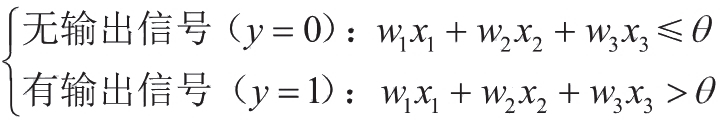# 深度学习的数学 (2)：神经网络的思想 1-2(神经元工作的数学表示)

《深度学习的数学》基于丰富的图示和具体示例，通俗易懂地介绍了深度学习相关的数学知识。第 1 章介绍神经网络的概况；第 2 章介绍理解神经网络所需的数学基础知识；第 3 章介绍神经网络的 * 优化；第 4 章介绍神经网络和误差反向传播法；第 5 章介绍深度学习和卷积神经网络。书中使用 Excel 进行理论验证，帮助读者直观地体验深度学习的原理。

### 整理神经元的工作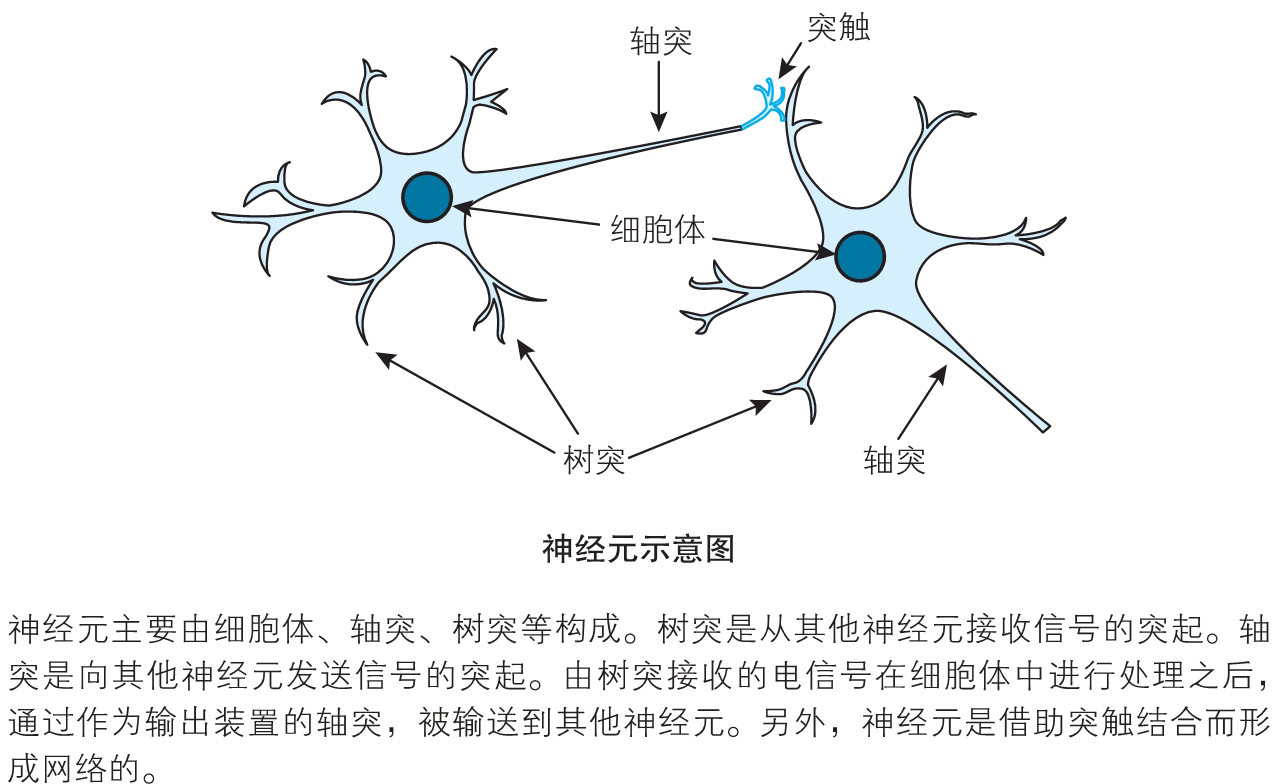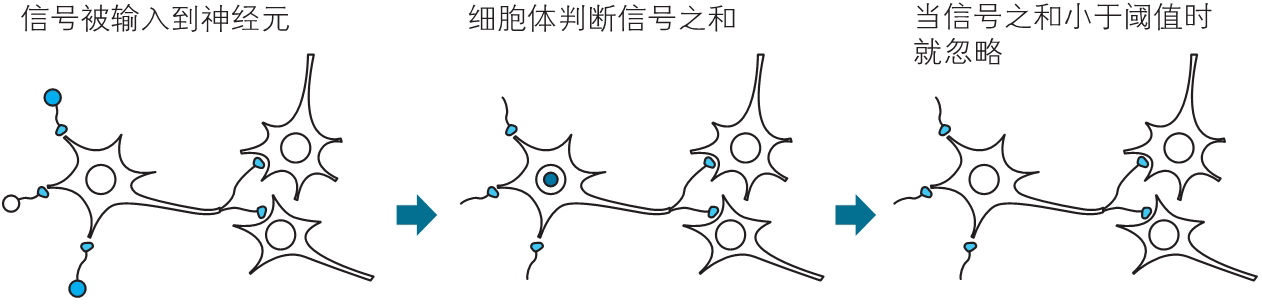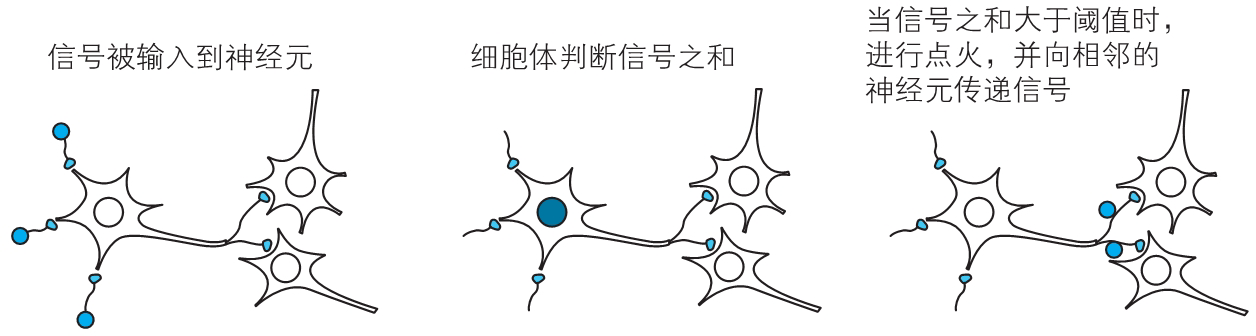### 神经元工作的数学表示

(i)　来自其他多个神经元的信号之和成为神经元的输入。

(ii)　如果这个信号之和超过神经元固有的阈值，则点火。

(iii)　神经元的输出信号可以用数字信号 0 和 1 来表示。即使有多个输出端，其值也是同一个。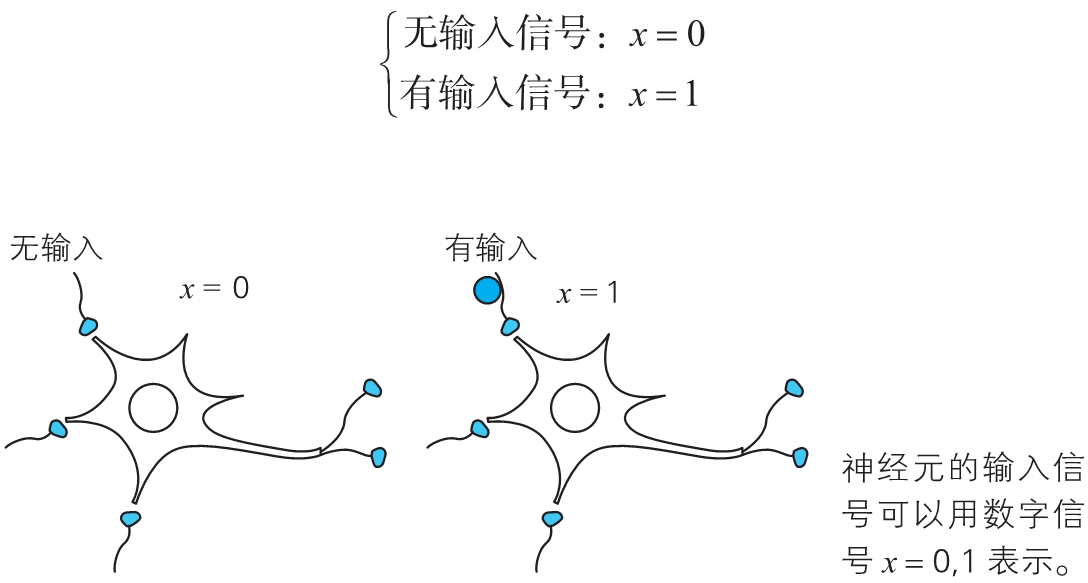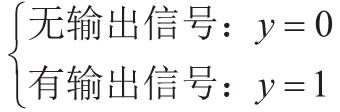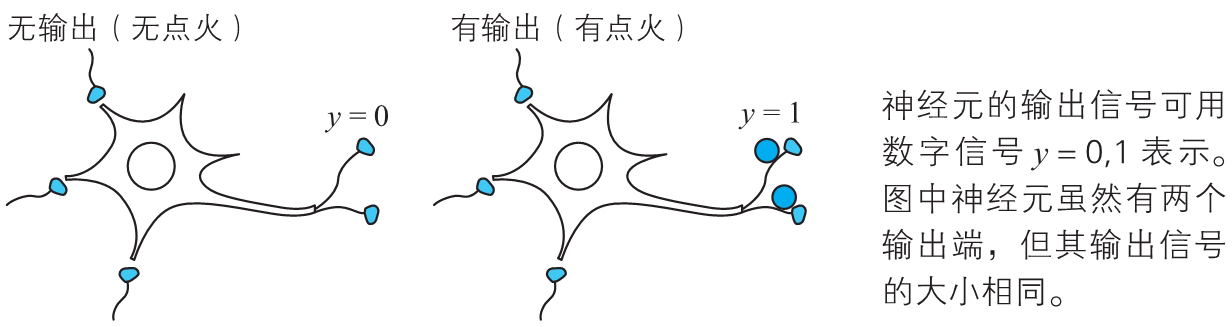$w_1x_1+w_2x_2+w_3x_3\quad\quad\quad\quad\quad\quad\quad\quad(1)$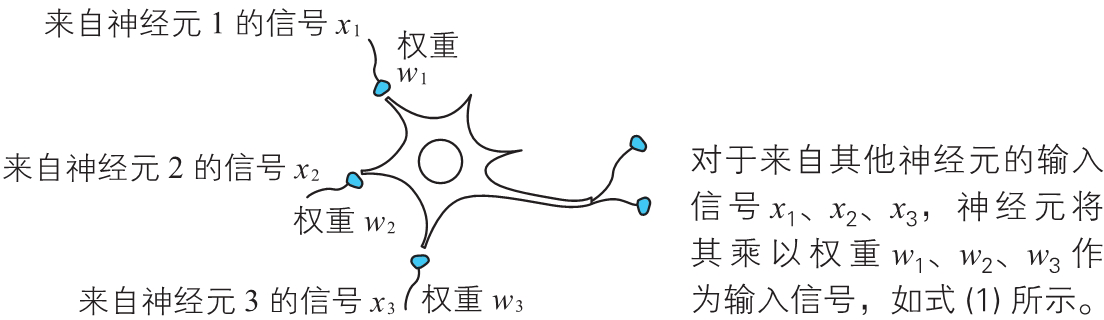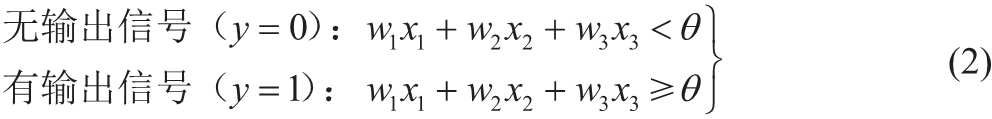0 0 $5\times0+3\times0=0<4$ 0
0 1 $5\times0+3\times1=3<4$ 0
1 0 $5\times1+3\times0=5\geqslant4$ 1
1 1 $5\times1+3\times1=8\geqslant4$ 1

### 点火条件的图形表示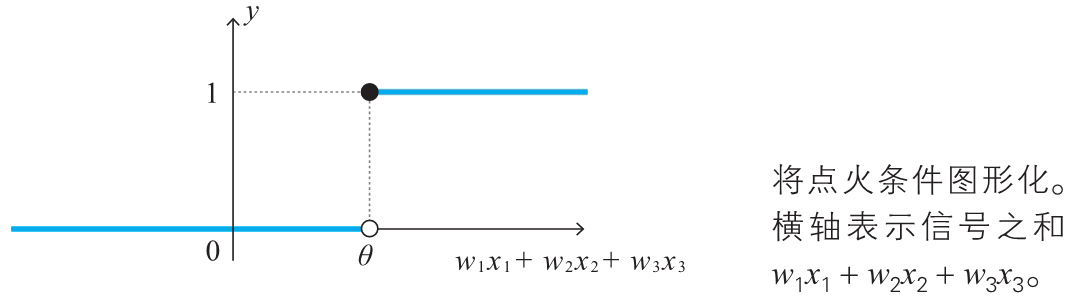$u(z)=\begin{cases}0~~(z<0)\1~~(z\geqslant0)\end{cases}$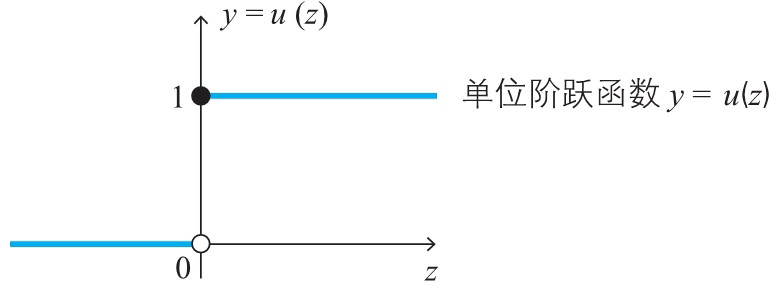|$\boldsymbol{y}$|$\boldsymbol{w_1x_1+w_2x_2+w_3x_3}$|$\boldsymbol{z=w_1x_1+w_2x_2+w_3x_3}-\theta$|$\boldsymbol{u(z)}$|
|-|-|
|0（无点火）|小于 $\theta$|$z<0$|0|
|1（点火）|大于等于 $\theta$|$z\geqslant0$|1|

$z=w_1x_1+w_2x_2+w_3x_3-\theta\quad\quad\quad\quad\quad\quad\quad\quad(4)$